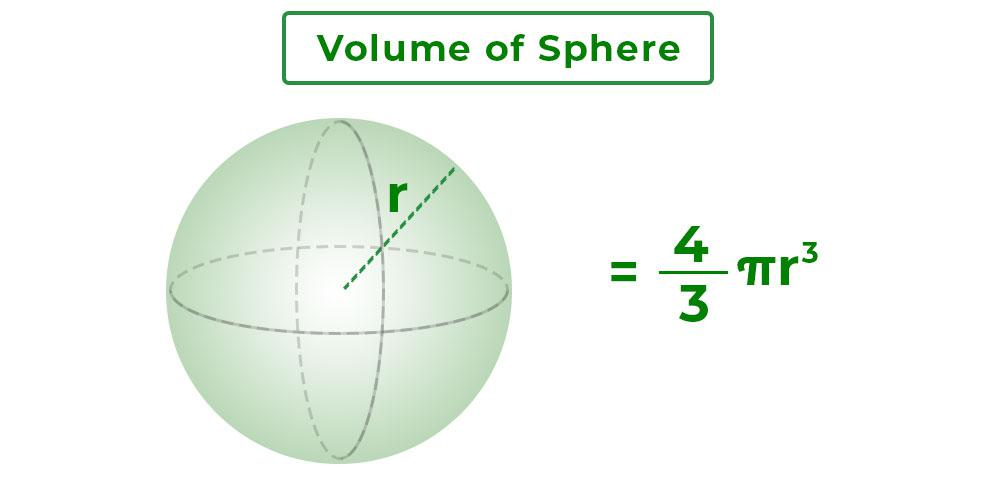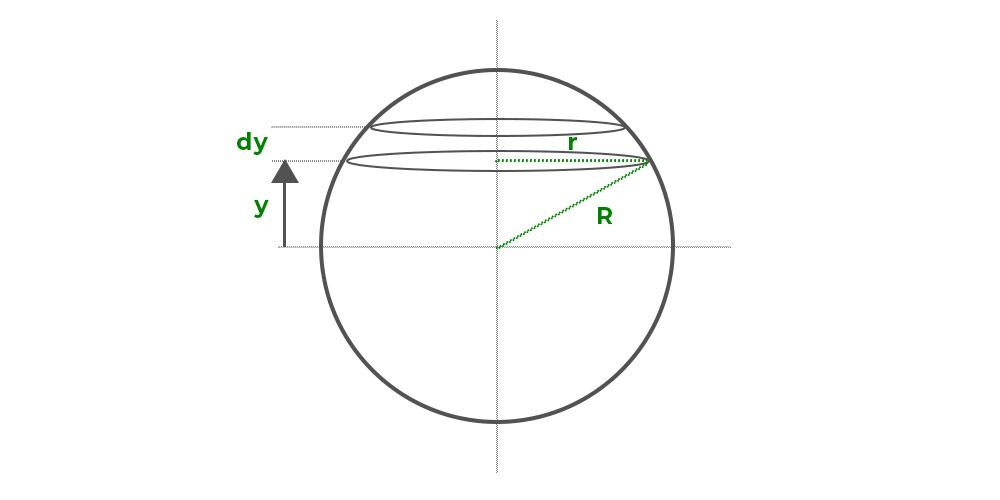Open in App
Not now

# Volume of a Sphere

• Last Updated : 10 Aug, 2022

The volume of sphere is the amount of liquid a sphere can hold. It is the space occupied by a sphere in 3-dimensional space. It is measured in unit3 i.e. m3, cm3, etc.

A sphere is a three-dimensional solid object with a round form in geometry. From a mathematical standpoint, it is a three-dimensional combination of a group of points connected by one common point at equal distances. A sphere, unlike other three-dimensional shapes, has no vertices or edges. Its centre is equidistant from all places on its surface. In other words, the distance between the sphere’s centre and any point on its surface is the same. Various spherical objects used in daily life are football, basketball, Earth, Moon, etc.

## What is Volume of Sphere?

The volume of a sphere is the amount of space it takes up within it. The sphere is a three-dimensional round solid shape in which all points on its surface are equally spaced from its centre. The fixed distance is known as the sphere’s radius, and the fixed point is known as the sphere’s centre. We will notice a change in form when the circle is turned. As a result of the rotation of the two-dimensional object known as a circle, the three-dimensional shape of a sphere is obtained.The formula for the volume of a sphere is given by,

V = 4/3πr3

where,

r = radius of the sphere

π = 22/7

## Volume of Sphere Formula with its Derivation

Using the integration approach, we can simply calculate the volume of a sphere.Suppose the sphere’s volume is made up of a series of thin circular discs stacked one on top of the other, as drawn in the diagram above. Each thin disc has a radius of r and a thickness of dy that is y distance from the x-axis.

Let the volume of a disc be dV. The value of dV is given by,

dV = (πr2)dy

dV = π (R2 – y2)dy

The total volume of the sphere will be the sum of volumes of all these small discs. The required value can be obtained by integrating the expression from limit -R to R.

So, the volume of sphere becomes,

V =Thus, the formula for volume of sphere is derived.

## How to Calculate Volume of Sphere?

Volume of sphere is the space occupied by a sphere. Its volume can be calculated using the formula  V = 4/3πr3. Steps required to calculate the volume of a sphere are:

1. Mark the value of the radius of the sphere.
2. Find the cube of the radius.
3. Multiply the cube of the radius by (4/3)π

Example: Find the volume of a sphere with a radius of 7 cm.

Solution:

The formula for volume of a sphere, V = (4/3)πr3
Given, r = 7 cm
Volume of sphere, V = ((4/3) × π × 73) cm3
V = 1436.8 cm3
Thus, the volume of sphere is 1436.8 cm3

## Solved Examples on Volume of Sphere

Example 1. Find the volume of the sphere whose radius is 9 cm.

Solution:

We have, r = 9.

Volume of sphere = 4/3 πr3

= (4/3) (3.14) (9) (9) (9)

= (4) (3.14) (3) (9) (9)

= 3052 cm3

Example 2. Find the volume of the sphere whose radius is 12 cm.

Solution:

We have, r = 12

Volume of sphere = 4/3 πr3

= (4/3) (3.14) (12) (12) (12)

= (4) (3.14) (4) (12) (12)

= 7234.56 cm3

Example 3. Find the volume of the sphere whose radius is 6 cm.

Solution:

We have, r = 6.

Volume of sphere = 4/3 πr3

= (4/3) (3.14) (6) (6) (6)

= (4) (3.14) (2) (6) (6)

= 904.32 cm3

Example 4. Find the volume of the sphere whose radius is 4 cm.

Solution:

We have, r = 4.

Volume of sphere = 4/3 πr3

= (4/3) (3.14) (4) (4) (4)

= (1.33) (3.14) (4) (4) (4)

= 267.27 cm3

Example 5. Find the volume of the sphere whose diameter is 10 cm.

Solution:

We have, 2r = 10

=> r = 10/2

=> r = 5

Volume of sphere = 4/3 πr3

= (4/3) (3.14) (5) (5) (5)

= (1.33) (3.14) (5) (5) (5)

= 522.025 cm3

Example 6. Find the volume of the sphere whose diameter is 16 cm.

Solution:

We have, 2r = 16

=> r = 16/2

=> r = 8

Volume of sphere = 4/3 πr3

= (4/3) (3.14) (8) (8) (8)

= (1.33) (3.14) (8) (8) (8)

= 2138.21 cm3

Example 7. Find the volume of the sphere whose diameter is 14 cm.

Solution:

We have, 2r = 14

=> r = 14/2

=> r = 7

Volume of sphere = 4/3 πr3

= (4/3) (3.14) (7) (7) (7)

= (1.33) (3.14) (7) (7) (7)

= 1432.43 cm3

## FAQs on Volume of Sphere

Question 1: Write the formula for the total surface area of the sphere.

Total surface area of any sphere is given by:

Area = 4πr2

where ‘r’ is the radius of the given sphere.

Questions 2: What is the formula for the Volume of the Sphere?

The volume of a sphere is given by:

Volume = 4/3πr3

where ‘r’ is the radius of the given sphere.

Question 3: How do we find the volume of Hemi-Sphere?

The volume of a hemi-sphere is given by:

Volume = 2/3πr3

where ‘r’ is the radius of the given sphere.

Question 4: If a sphere and a hemisphere have the same radii then what is the ratio of their volume?

If a sphere and a hemisphere have the same radii then the ratio of their volume is given by

V1 : V2 (4/3πr3) : (2/3πr3)

= 2 : 1

Question 5: How do we measure the volume of Sphere?